American Journal of Theoretical and Applied Statistics
Volume 5, Issue 1, January 2016, Pages: 5-12

Review Article

A Brief Review of Tests for Normality

Keya Rani Das1, *, A. H. M. Rahmatullah Imon2

1Department of Statistics, Bangabandhu Sheikh Mujibur Rahman Agricultural University, Gazipur, Bangladesh

2Department of Mathematical Sciences, Ball State University, Muncie, IN, USA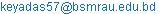(K. R. Das)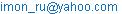(A. H. M. R. Imon)

Keya Rani Das, A. H. M. Rahmatullah Imon. A Brief Review of Tests for Normality. American Journal of Theoretical and Applied Statistics. Vol. 5, No. 1, 2016, pp. 5-12. doi: 10.11648/j.ajtas.20160501.12

Abstract: In statistics it is conventional to assume that the observations are normal. The entire statistical framework is grounded on this assumption and if this assumption is violated the inference breaks down. For this reason it is essential to check or test this assumption before any statistical analysis of data. In this paper we provide a brief review of commonly used tests for normality. We present both graphical and analytical tests here. Normality tests in regression and experimental design suffer from supernormality. We also address this issue in this paper and present some tests which can successfully handle this problem.

Keywords: Power, Empirical Cdf, Outlier, Moments, Skewness, Kurtosis, Supernormality

1. Introduction

In all branches of knowledge it is necessary to apply statistical methods in a sensible way. In the literature statistical misconceptions are conventional. The most commonly used statistical methods are correlation, regression and experimental design. But all of them are based on one basic assumption, that the observation follows normal (Gaussian) distribution. So it is assumed that the populations from where the samples are collected are normally distributed. For this reason the inferential methods require checking the normality assumption.

In the last hundred years, attitudes towards the assumption of a Normal distribution in statistical models have varied from one extreme to another. To quote Pearson (1905) ‘Even towards the end of the nineteenth century not all were convinced of the need for curves other than normal.’ By the middle of this century Geary (1947) made this comment `Normality is a myth; there never was and never will be a normal distribution.' This might be an overstatement, but the fact is that non-Normal distributions are more prevalent in practice than formerly assumed.

Gnanadesikan (1977) pointed out, `the effects on classical methods of departure from normality are neither clearly nor easily understood.' Nevertheless, evidence is available that shows such departures can have unfortunate effects in a variety of situations. In regression problems, the effects of departure from normality in estimation were studied by Huber (1973). He pointed out that, under non-Normality it is difficult to find necessary and sufficient conditions such that all estimates of the parameters are asymptotically normal. In testing hypotheses, the effect of departure from normality has been investigated by many statisticians. A good review of these investigations is available in Judge et al. (1985). When the observations are not normally distributed, the associated normal and chi-square tests are inaccurate and consequently the t and F tests are not generally valid in finite samples. However, they have an asymptotic justification. The sizes of t and F tests appear fairly robust to deviation from normality [see Pearson and Please (1975)]. This robustness of validity is obviously an attractive property, but it is important to investigate the response of tests' power as well as size to departure from normality. Koenker (1982) pointed out that the power of t and F tests is extremely sensitive to the hypothesized distribution and may deteriorate very rapidly as the distribution becomes long-tailed. Furthermore, Bera and Jarque (1982) have found that homoscedasticity and serial independence tests suggested for normal observations may result in incorrect conclusions under non-normality. It may be also essential to have proper knowledge of observations in prediction and in confidence limits of predictions. Most of the standard results of this particular study are based on the normality assumption and the whole inferential procedure may be subjected to error if there is a departure from this. In all, violation of the normality assumption may lead to the use of suboptimal estimators, invalid inferential statements and inaccurate predictions. So for the validity of conclusions we must test the normality assumption. The main objective of this paper is to accumulate the procedures by which we can examine normality assumption. There is now a very large body of literature on tests for Normality and many textbooks contain sections on the topic. Mardia (1980) and D'Agostino (1986) gave excellent reviews of these tests. We consider in this paper a few of them which are selected mainly for their good power properties. The prime objective of this paper is to distinguish different types of normality tests for different areas of statistics. For the moment practitioners indiscriminantly apply normality tests. But in this paper we will show tests developed for univariate independent samples should not be readily applied for regression and design of experiments because of the supernormality problem. We try to categorize the normality tests in several classes although we recognize the fact that there are many more tests (not considered here) which may not come under these categories. This consists of both graphical plots and analytical test procedures.

2. Graphical Method

Any statistical analysis enriched by including appropriate graphical checking of the observation. To quote Chambers et al. (1983) ‘Graphical methods provide powerful diagnostic tools for confirming assumptions, or, when the assumptions are not met, for suggesting corrective actions. Without such tools, confirmation of assumptions can be replaced only by hope.’ Some statistical plots such as scatter plots, residual plots are advised for checking or diagnostic statistical method. For goodness of fit and distribution curve fitting graphical plots are necessary and give ideas about pattern. Existing testing methods give an objective decision of normality. But these do not provide general hint about cause of rejecting a null hypothesis. So, we are interested to present different types plot for normality checking as well as various testing procedures of it. Generally histograms, stem-and-leaf plots, box plots, percent-percent (P-P) plots, quantile-quantile (Q-Q) plots, plots of the empirical cumulative distribution function and other variants of probability plots have most application for normality assumption checking.

2.1. Histogram

The easiest and simplest graphical plot is the histogram. The frequency distribution in which the observed values are plotted against their frequency, states a visual estimation whether the distribution is bell shaped or not. At the same, time it provides indication about insights gap in the data and outliers. Also it gives idea about skewness or symmetry.

Data that can be represented by this type of ideal, bell-shaped curve as shown in the first graph are said to have a normal distribution or to be normally distributed. Of course for the second graph the data are not normally distributed.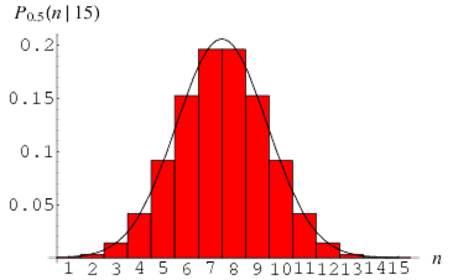Figure 1. Histogram shows the data are normally distributed.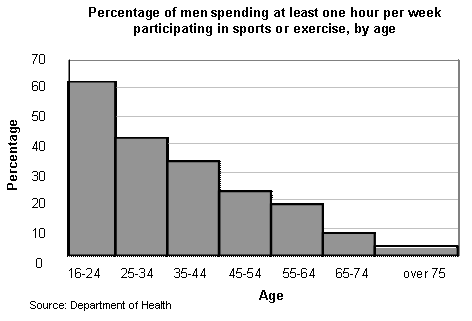Figure 2. Histogram shows the data are not normally distributed.

2.2. Stem-and-Leaf Plot

Stem-and-leaf display states identical knowledge as like histogram but observation appeared with their identity seems they do not lose their any information about original data. Like histogram, they show frequency of observations along with median value, highest and lowest values of the distribution, other sample percentiles from the display of data. There is a "stem" and a "leaf" for each values where the stems depicts a set of bins in which leaves are grouped and these leaves reflect bars like histogram.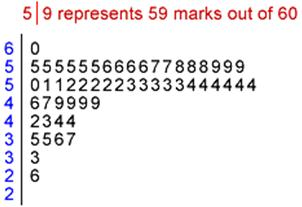Figure 3. Stem-and-leaf plot shows the data are not normally distributed.

The above stem-and-leaf plot of marks obtained by the students clearly shows that the data are not normally distributed.

2.3. Box-and-Whisker Plot

It has another name as five number summary where it needs first quartile, second quartile or median, third quartile, minimum and maximum values to display. Here we try to plot our data in a box whose midpoint is the sample median, the top of the box is the third quartile (Q3) and the bottom of the box is the first quartile (Q1). The upper whisker extends to this adjacent value - the highest data value within the upper limit = Q3 + 1.5 IQR where the inter quartile range IQR is defined as IQR = Q3-Q1. Similarly the lower whisker extends to this adjacent value - the lowest value within the lower limit = Q1- 1.5 IQR.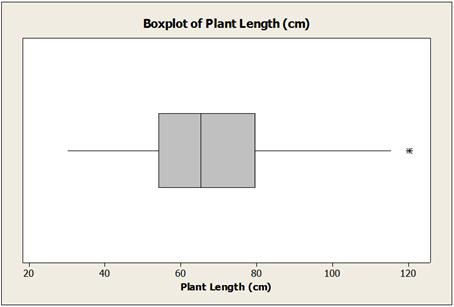Figure 4. Box-and-Whisker plot shows the data are not normally distributed.

We consider an observation to be unusually large or small when it is plotted beyond the whiskers and they are treated as outliers. By this plot we can get clear indication about symmetry of data set. At the same time it gives idea about scatteredness of observations. Thus the normality pattern of the data is understood by this plot as well.

The box plot presented in Figure 1 is taken from Imon and Das (2015). This plot clearly shows non-normal pattern of the data. It contains outlier and the data are not even symmetric which is, in fact, skewed to the right.

2.4. Normal Percent-Percent Plot

In statistics, a P–P plot (probability–probability plot or percent–percent plot) is a probability plot for assessing how closely two data sets agree, which plots the two cumulative distribution functions against each other. From this plot we get idea about outlier, skewnwss, kurtosis and for this reason it has become a very popular tool for testing the normality assumption.

A P-P plot compares the empirical cumulative distribution function of a data set with a specified theoretical cumulative distribution function F(·). If it looks like straight line or there is no curve then it contains no outliers and the assumption thought to be fulfilled and if it shows another outlook than straight line (e. g. curve), the assumption surmised to be failed.

Normal P-P plots presented in Figures 5 and 6 are taken from Imon (2015). The first plot shows a normality pattern and the second one exhibits non-normality and the existence of an outlier.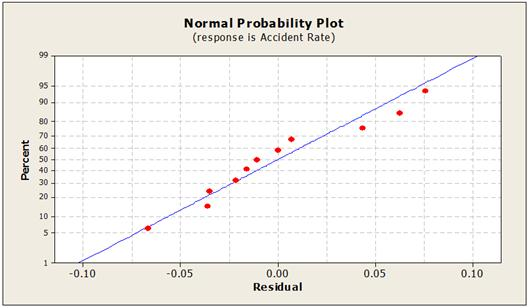Figure 5. Normal Percent-percent plot shows the data are normally distributed.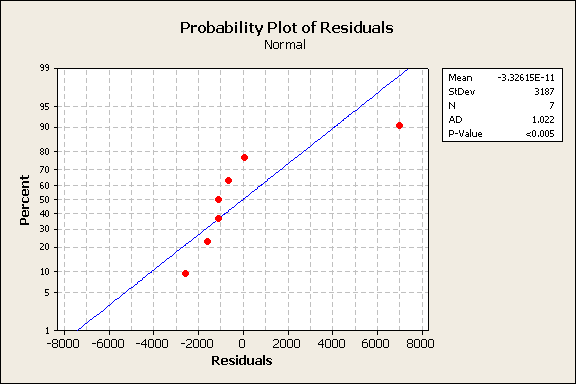Figure 6. Normal percent-percent plot shows the data are non-normal.

2.5. Normal Quantile-Quantile Plot

A quantile-quantile(Q-Q)plot compares the quantiles of a data distribution with the quantiles of a standardized theoretical distribution from a specified family of distributions. A normal Q-Q plot is that which we can shaped by plotting quantiles of one distribution versus quantiles of normal distribution. When quantiles of two distributions are met, plotted dots face with the line y = x. If it shows curve size with slope rising from left to right, it indicates the data distribution is skewed to the right and curve size with slope decreasing from left to right, it exposes skewness is to the left for the distribution. By investigating in normal probability paper, a Q-Q plot can easily be produced by hand. The abscissa on probability paper is scaled in proportionally to the expected quantiles of a standard normal distribution so that a plot of (p,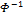(p)) is linear. The abscissa limits typically run from 0.0001 to 0.9999. The vertical scale is linear and does not require that the data be standardized in any manner; also available is probability paper that is scaled logarithmically on the y-axis for use in determining whether data is lognormally distributed. On probability paper, the pairs (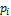,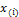) are plotted. For plots done by hand, the advantage of Q-Q plots done on normal probability paper is that percentiles and cumulative probabilities can be directly estimated, and,() need not be obtained to create the plot.

There is a great area of confusion between P-P plot and Q-Q plot and sometimes people think that they are synonymous. But there are three important differences in the way P-P plots and Q-Q plots are constructed and interpreted:

The construction of a Q-Q plot does not require that the location or scale parameters of F(·) be specified. The theoretical quantiles are computed from a standard distribution within the specified family. A linear point pattern indicates that the specified family reasonably describes the data distribution, and the location and scale parameters can be estimated visually as the intercept and slope of the linear pattern. In contrast, the construction of a P-P plot requires the location and scale parameters of F(·) to evaluate the cdf at the ordered data values.

The linearity of the point pattern on a Q-Q plot is unaffected by changes in location or scale. On a P-P plot, changes in location or scale do not necessarily preserve linearity.

On a Q-Q plot, the reference line representing a particular theoretical distribution depends on the location and scale parameters of that distribution, having intercept and slope equal to the location and scale parameters. On a P-P plot, the reference line for any distribution is always the diagonal line y = x.

Consequently, you should use a Q-Q plot if your objective is to compare the data distribution with a family of distributions that vary only in location and scale, particularly if you want to estimate the location and scale parameters from the plot.

An advantage of P-P plots is that they are discriminating in regions of high probability density, since in these regions the empirical and theoretical cumulative distributions change more rapidly than in regions of low probability density. For example, if you compare a data distribution with a particular normal distribution, differences in the middle of the two distributions are more apparent on a P-P plot than on a Q-Q plot.

2.6. Empirical Cumulative Distribution Function Plot

An empirical CDF plot performs a similar function as a probability plot. However, unlike a probability plot, the empirical CDF plot has scales that are not transformed and the fitted distribution does not form a straight line, rather it yields an S-shape curve under normality. The empirical cumulative probabilities close to this S-shape curve satisfies the normality assumption.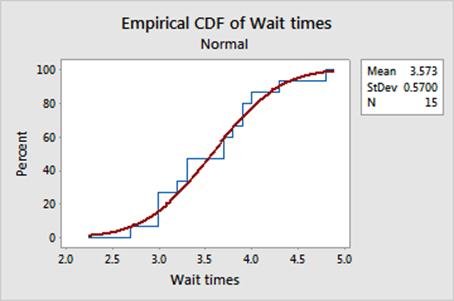Figure 7. Empirical cumulative distribution function plot shows the data are normally distributed.

2.7. Detrended Probability Plot

In statistics, a graph of the differences between observed and expected values, the expected values being based on the assumption of a normal distribution. If the observed scores are normally distributed, then the points should cluster in a horizontal band close to zero without any discernible pattern. This is also known as the detrended Q-Q plot since here (-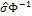()) is plotted against the plotting positionor the expected quantile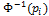for some estimate of the standard deviation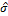. If the observations come from a normal distribution, the result should be a straight line with zero slope.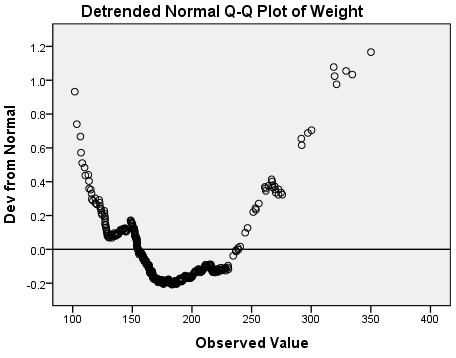Figure 8. Detrended normal Q-Q plot.

3. Analytical Test Procedures

Various types of descriptive measures like moments, cumulants, coefficients of skewness and kurtosis, mean deviation, range of the sample etc. and empirical distribution function have been proposed for use in tests for normality, but only a few of them are frequently used in practice. Here we categorize tests into two groups: tests based on empirical distribution function (EDF) test and tests based on descriptive measures.

3.1. Empirical Distribution Function (EDF) Tests

Based on the measure of discrepancy between empirical and hypothesized distributions generally mentioned as empirical distribution function we can define the following tests.

3.1.1. Kolmogorov-Smirnov Test

The Kolmogorov-Smirnov test was first derived by Kolmogorov (1933) and later modified and proposed as a test by Smirnov (1948). The test statistic is(1)

where, F(X, µ, σ) is the theoretical cumulative distribution function of the normal distribution function and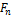(X)is the empirical distribution function of the data. If it gives large values of D then it indicates the data are not normal. When population parameters (µ and σ) are unknown then sample estimates are used instead of parameter values.

3.1.2. Shapiro-Wilk Test

The Shapiro-Wilk test is one of the most popular tests for normality assumption diagnostics which has good properties of power and it based on correlation within given observations and associated normal scores. The Shapiro-Wilk test statistic is derived by Shapiro and Wilk (1965). The form of the test statistic is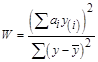(2)

where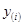is the i-th order statistics and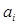is the i-th expected value of normalized order statistics. For independently and identically distributed observations, the values ofcan be obtained from the table presented by Shapiro and Wilk (1965) for sample sizes up to 50. W can be expressed as a square of the correlation coefficient betweenand. So W is location and scale invariant and is always less than or equal to 1. In the plot ofagainstan exact straight line would lead to W very close to 1. So if W is significantly less than 1, the hypothesis of Normality will be rejected.

Although the Shapiro-Wilk W test is very popular, it depends on availability of values of, and for large sample cases their computation may be much more complicated. Some minor modifications to the W test have been suggested by Shapiro and Francia (1972), Weisberg and Bingham (1975) and Royston (1982). An alternative test of the same nature for samples larger than 50 is designed by D'Agostino (1971).

3.1.3. Anderson-Darling Test

Stephens (1974) proposed a test based on empirical distribution by extending the work of Anderson and Darling (1952). This test is generally known as Anderson-Darling normality test. For normally distributed random observationswith mean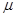and variance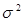, the Anderson-Darling test statistic is given by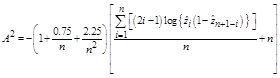(3)

Where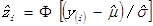and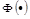is the distribution function of an N (0,1) random variable. Stephens (1974) provided the percentage points for this test.

3.2. Tests Based on Descriptive Measures

Fisher (1930) proposed using cumulants. Using his result, Pearson (1930) obtained the first four moments of the sampling distribution of skewness and kurtosis, under the null hypothesis of normality. He used those results to develop criteria for testing normality by using sample values of coefficients of skewness and kurtosis separately. The ratio of mean deviation to standard deviation [see Geary (1935)] and ratio of sample range to standard deviation [see David, Hartley, and Pearson (1954)] were also proposed for the same purpose. Based on moments the most popular tests are D’Agostino-Pearson Omnibus test and Jarqua-Bera test.

3.2.1. D’Agostino-Pearson Omnibus Test

To assessing the symmetry or asymmetry generally skewness is measured and to evaluate the shape of the distribution kurtosis is overlooked. D’Agostino-Pearson (1973) test standing on the basis of skewness and kurtosis test and these are also assessing through moments. The DAP statistic is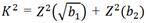(4)

where Z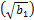and Z(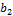) are the normal approximation equivalent to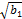andare sample skewness and kurtosis respectively. This statistic follows a chi-squared distribution with two degrees of freedom if the population is from normal distribution. A large value of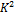leads to the rejection of the normality assumption.

3.2.2. Jarqua-Bera Test

The Jarqua-Bera test was originally proposed by Bowman and Shenton (1975). They combined squares of normalized skewness and kurtosis in a single statistic as follows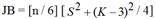(5)

This normalization is based on normality since S = 0 and K = 3 for a normal distribution and their asymptotic variances are 6/n and 24/n respectively. Hence under normality the JB test statistic follows also a chi-squared distribution with two degrees of freedom. A significantly large value of JB leads to the rejection of the normality assumption.

4. Supernormality and Rescaled Moments Test

Test procedures discussed so far can be applied for testing normality of the distribution from which we have collected the observations. Here the normality test is employed on an observed data set. But in regression and design problems, since the true errors are unobserved, it is a common practice to use the residuals as substitutes for them in tests for normality. The residuals have several drawbacks which have made statisticians question [see Cook and Weisberg (1982)] whether they can be used as proper substitutes for the true errors or not. In testing normality, all test statistics have been designed on the basis of independent and identically distributed random observations. An immediate problem of using residuals in them is that even when the true errors are independent, their corresponding residuals are always correlated. Residuals also have the problem of not possessing constant variance while the true errors do so. They also have the disadvantage that their probability distribution is always closer to normal form than is the probability distribution of the true errors, when the errors are not normal. This problem is generally known as the supernormality effect of the residuals.

Since the question has been raised about the use of residuals as proper estimates of the errors because of supernormality, this practice of using them in test procedures looks questionable. But, most important, the induced normality of the residuals makes a test of normality of the true errors based on residuals logically very weak.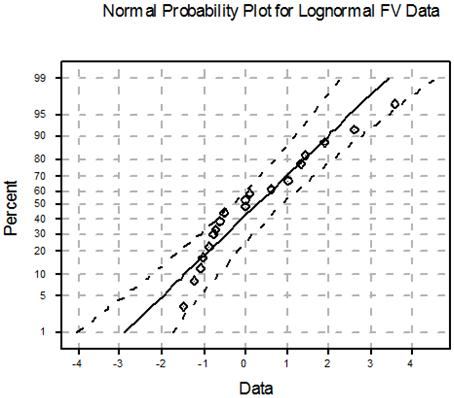Figure 9. Normal probability plot for lognormal data.

The above graph is taken from Imon (2003a). Although the true errors are lognormal, the corresponding residuals accept normality. Because of this effect most of the normality tests based on residuals possess very poor power. To overcome this problem Imon (2003b) suggests a slight adjustment to the JB statistic to make it more suitable for the regression problems. His proposed statistic based on rescaled moments (RM) of ordinary least squares residuals is defined as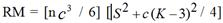(6)

where c = n/(n – k), k is the number of independent variables in a regression model. Both the JB and the RM statistic follow a chi square distribution with 2 degrees of freedom. If the values of these statistics are greater than the critical value of the chi square, we reject the null hypothesis of normality. Rana, Habshah, and Imon (2009) proposed a robust version of the RM test for regression and design of experiments.

5. Conclusions

It is essential to assess normality of a data before any formal statistical analysis. Otherwise we might draw erroneous inference and wrong conclusions. Normality can be assessed both visually and through normality tests. Most of the statistical packages automatically produce the PP and QQ plots. Since graphical tests are very much subjective use of analytical test is highly recommended. Among the analytical tests the Shapiro-Wilk test is provided by the SPSS software and possesses very good power properties. However, the Jarque-Bera test has become more popular to the practitioners especially in economics and business. But both Shapiro-Wilk and Jarque-Bera tests are not appropriate when we test normality of residuals in regression and/or design of experiments. We recommend using the rescaled moments test in this regard.

References

1. Anderson, T.W., and Darling, D.A. 1952. ‘‘Asymptotic theory of certain goodness-of-fit criteria based on stochastic processes.’’ The Annals of Mathematical Statistics 23(2): 193-212. http://www.cithep.caltech.edu/~fcp/statistics/hypothesisTest/PoissonConsistency/AndersonDarling1952.pdf.
2. Bera, A.K., and Jarque, C.M. 1982. ‘‘Model specification tests: A simultaneous approach.’’ Journal of Econometrics 20: 59-82.
3. Bowman, K.O., and Shenton, B.R. 1975. ‘‘Omnibus test contours for departures from normality based onand.’’Biometrika 64: 243-50.
4. Chambers, J.M., Cleveland, W.S., Kleiner, B., and Tukey, P.A. 1983. Graphical Methods for Data Analysis. Boston. Duxbury Press.
5. Cook, R.D., and Weisberg, S. 1982. Residuals and Influence in Regression. New York. Chapman and Hall.
6. D'Agostino, R.B. 1971. ‘‘An omnibus test of normality for moderate and large sample sizes.’’ Biometrika 58(August): 341-348.
7. D'Agostino, R.B. 1986. ‘‘Tests for normal distribution.’’ In Goodness-of-fit Techniques, edited by D'Agostino, R.B., and Stephens, M.A. 367-420. New York. Marcel Dekker.
8. DʼAgostino R, and Pearson E. S. 1973. ‘‘Tests for departure from normality. Empirical results for the distributions ofand’’ Biometrika. 60(3), 613-622.
9. David, H. A., Hartley, H. O., and Pearson, E. S. 1954. ‘‘The distribution of the ratio, in a single normal sample of range to standard deviation.’’ Biometrika 41: 482-93.
10. Fisher, R. A. 1930. ‘‘The moments of the distribution for normal samples of measures of departure from normality.’’ Proceedings of the Royal Society of London 130(December): 16-28.
11. Geary, R. C. 1935. ‘‘The ratio of mean deviation to the standard deviation as a test of normality.’’ Biometrika 27: 310-332.
12. Geary, R. C. 1947. ‘‘Testing for normality.’’ Biometrika 34: 209-242.
13. Gnanadesikan, R. 1977. Methods for Statistical Analysis of Multivariate Data. New York. Wiley.
14. Huber, P. J. 1973. ‘‘Robust regression: Asymptotics, conjectures, and Monte Carlo.’’ The Annals of Statistics 1(5): 799-821. DOI: 10.1214/aos/1176342503.
15. Imon, A. H. M. R. 2003. ‘‘Simulation of errors in linear regression: An approach based on fixed percentage area.’’Computational Statistics 18(3): 521–531.
16. Imon, A. H. M. R. 2003. ‘‘Regression residuals, moments, and their use in tests for normality.’’Communications in Statistics—Theory and Methods,32(5): 1021–1034.
17. Imon, A. H. M. R. 2015. ‘‘An Introduction to Regression, Time Series, and Forecasting.’’ (To appear).
18. Imon, A. H. M. R., and Das, K. 2015. ‘‘Analyzing length or size based data: A study on the lengths of peas plants.’’ Malaysian Journal of Mathematical Sciences 9(1): 1-20. http://einspem.upm.edu.my/journal/fullpaper/vol9/1.%20imon%20&%20keya.pdf.
19. Judge, G. G., Griffith, W. E., Hill, R. C., Lutkepohl, H., and Lee, T. 1985. Theory and Practice of Econometrics. 2nd. Ed. New York. Wiley.
20. Koenker, R. W. 1982. ‘‘Robust methods in econometrics.’’ Econometric Reviews 1: 213-290.
21. Kolmogorov, A. 1933. ‘‘Sulla determinazione empirica di una legge di distribuzione.’’G. Ist. Ital. Attuari 4, 83–91.
22. Mardia, K. V. 1980. ‘‘Tests of univariate and multivariate normality.’’ In Handbook of Statistics 1: Analysis of Variance, edited by Krishnaiah, P. R. 279-320. Amsterdam. North-Holland Publishing.
23. Pearson, K. 1905. ‘‘On the general theory of skew correlation and non-linear regression.’’ Biometrika 4: 171-212.
24. Pearson, E. S. 1930. ‘‘A further development of tests for normality.’’ Biometrika 22(1-2): 239-249. doi: 10.1093/biomet/22.1-2.239.
25. Pearson, E. S., and Please, N. W. 1975. ‘‘Relation between the shape of population distribution and the robustness of four simple statistical tests.’’ Biometrika 62: 223-241.
26. Rana, M. S., Habshah, M. and Imon, A. H. M. R. 2009. ‘‘A robust rescaled moments test for normality in regression.’’ Journal of Mathematics and Statistics 5 (1): 54–62.
27. Royston, J. P. 1982. ‘‘An extension of Shapiro-Wilk's W test for non-normality to large samples.’’ Applied Statistics 31: 115-124.
28. Shapiro, S. S., and Francia, R. S. 1972. ‘‘An approximate analysis of variance test for normality.’’ Journal of the American Statistical Association 67(337): 215-216. DOI:10.1080/01621459.1972.10481232.
29. Shapiro, S. S., and Wilk, M. B. 1965. ‘‘An analysis of variance test for normality (complete samples).’’ Biometrika 52(3/4): 591-611. http://sci2s.ugr.es/keel/pdf/algorithm/articulo/shapiro1965.pdf.
30. Smirnov, N. 1948. ‘‘Table for estimating the goodness of fit of empirical distributions.’’ Annals of Mathematical Statistics 19(2): 279–281. doi:10.1214/aoms/1177730256.
31. Stephens, M. A. 1974. ‘‘EDF statistics for goodness of fit and some comparisons.’’ Journal of the American Statistical Association 69(347): 730-737.
32. Weisberg, S., and Bingham, C. 1975. ‘‘An approximate analysis of variance test for non-normality suitable for machine calculation.’’ Technometrics 17(1): 133-134.

 Contents 1. 2. 2.1. 2.2. 2.3. 2.4. 2.5. 2.6. 2.7. 3. 3.1. 3.2. 4. 5.
Article ToolsAbstractPDF(1460K)